www.batmath.it

### Saying maths 2 - Mainly numbers

#### Numbers: general remarks

• A point (.) is used for decimals, and not a comma (,); commas in figures are used only when writing thousands (example 10,000: ten thousand), but we prefer to avoid the use of commas in mathematical formulas.
• Use of "and"
• to indicate the location of the decimal point: so 100.5 is one hundred and 5 tenths, but one hundred point five is by far more common; 239.36 is two hundred thirty-nine and thirty-six hundredths, but two hundred thirty-nine point three six is by far more common;
• in British English we use and when saying numbers in the hundreds: 105 is one hundred and five;
• in American English we do not use and when saying numbers in the hundreds: 105 is one hundred five;
• anyhow we have also found on the Internet something like this: 105 is hundred five;
• so, as what is "correct" changes with time, choose your standard and follow it!
• The "0" (oh, nought, zero, love,  nil)
• oh
• after the decimal point: 27.05 is twenty-seven point oh five;
• in years: 1907 is nineteen oh seven;
• in telephone, bus, hotel room numbers (but this is not very important in maths!);
• nought
• before the decimal point: 0.05 is nought point oh five (but see also the next heading);
• tip: noughts and crosses is a game like the Italian "filetto";
• zero
• for the number 0 itself:  "0" is zero;
• before a decimal point, mainly in American English, but also in British English: 0.05 is zero point oh five, instead of the more "traditional" form "nought point oh five";
• for the temperature: -7°C is seven degrees below zero, which you can also say minus seven;
• nil
• in football scores: "Italy wins 3-0" is "Italy wins three nil" (also three to nil); sometimes nothing is used in the place of nil  "3-0" can be three to nothing;
• love
• in tennis games: "the score of the game is 15-0" is "the score of the game is fifteen love"; "0-0" is love all or love game.
• The decimal point
• the numbers after the decimal point are all read separately 0.0023 is nought point oh oh two three; use of "and" is also allowed (see the previous heading), but we prefer this way of saying numbers with a decimal point;
• you can also use shorter forms: 0.0005 can be read as nought point double oh oh five;
• in periodic numbers we use "recurring": 5.376666... is five point three seven `-pause-` six recurring, while 5.376376376... is five point three seven six `-pause-` all recurring;
• digits after the decimal point are (almost) always grouped while reading currencies, lengths, and other measures:
• £15.50 is fifteen pounds fifty / fifteen pounds fifty pence / fifteen fifty (if no misunderstanding can occur);
• €2.27 is two euro twenty-seven / two euro twenty-seven cents;
• 47.99s (seconds) is forty seven seconds ninety-nine hundredths;
• 3.87m (meters) is three meters eighty-seven centimetres.
• Kinds of numbers
• natural, whole, integer, rational, irrational, real, complex (imaginary)
• odd, even
• fractional
• prime
• random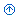#### Numbers: elementary calculations

 = The equals sign x=3 ;  x≠3 x equals three / x is equal to three  ;  x (is) not equal to three x≡y x is equivalent to (or identical with) y x>y  ; x≥y x is greater than y  ;  x is greater than or equal to y x> ; <<< ; >>> much less than ; much greater than ; very much less than ; very much greater than (The last two are not frequently used, but they are in the set of Unicode characters). a+b=s (addition) a and b are the addends, s is the sum, a and b are also the items of the addition. a+b=s a plus b is (/ equals / is equal to) s a and b is (/ equals  / is equal to) s s is the sum of a and b a-b=d (subtraction or difference) a is the minuend, b is the subtrahend, d is the remainder or the difference a-b=d a minus b is (/ equals / is equal to) d a take away b is (/ equals / is equal to) d d is the difference between a and b a±b a plus or minus b a×b=p, or a·b=p, or simply ab=p (multiplication) a and b are the factors or the multipliers, p is the product a×b=p, or a·b=p, or simply ab=p a times b is (/ equals / is equal to) p a multiplied by b is (/ equals / is equal to) p a by b is (/ equals / is equal to) p a b is (/ equals / is equal to) p p is the product of a and b a : b = q, or a / b = q (division) a is the dividend, b is the divisor, q is the quotient or the ratio a:b=q, or a/b=q a divided by b is (/ equals / is equal to) q q is the quotient of the division of a by b verbs concerning operations to sum, to subtract / to deduct, to multiply, to divide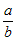(fraction) a is the numerator, b is the denominator (the outcome is always called the quotient, as in the division)a fraction can be said a divided by b (as a normal division ), or a over b. Cardinal numbers for the numerator and ordinal numbers for the denominator are also used (as in Italian):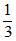is a third,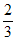is two thirds. Special cases are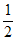(a/one half),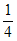(a/one quarter),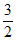(three halves),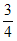(three quarters), and similar. The special notation sometimes used for improper fractions, as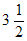, is said three and a half.

 |x| or abs(x) The absolute value of x ab a is the base, b is the index or the exponent x2 x squared / x (raised) to the power two x3 x cubed / x (raised) to the power three x4 x to the fourth / x (raised) to the power four xn x to the nth / x (raised) to the power n x-n x to the minus n / x (raised) to the power minus n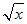root x / square root x / square root of x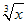cube root x / cube root of x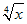fourth root x / fourth root of x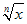nth root x / nth root of x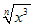nth root  `-pause-` x cubed or nth root   `-pause-` of x cubed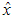x hat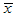x bar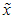x tilde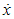x dot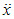x dot dot / x double dot n! n factorial / factorial n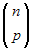n choose p xi x i / x subscript i / x suffix i / x sub i xi (not a power!) x index i / sometimes x i if no misunderstanding with xi can occur / x superscript i (x+y)3  ;  (x+y)n x plus y all cubed  ; x+y all to the nth x3+y3 x cubed plus y cubed a1 + a2 + ... +an a one plus a two and so on up to a (sub) n a1 × a2 × ... ×an a one times a two and so on up to a (sub) n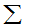the summation symbol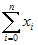the sum as i runs from zero to n of the x i / the sum from i equals zero to n of the x i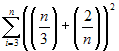the sum  `-pause-` as i runs from three to n  `-pause-` of the quantity n over 3  `-pause-` plus the quantity 2 over n  `-pause-` all squared (but probably nobody will understand what you mean if he can't read the blackboard or the transparency!!)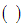parenthesis  -pl. parentheses / round brackets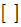brackets / square brackets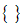braces / curly brackets π pi

#### Useful expressions

• the noughts and ones of computer language: to refer to the digits used by computers ("0" and "1")
• the slashed zero: the zero of the computer
• to reset to zero
• to extract a root
• to cast out nines (the famous test for divisions)
• to move the decimal point back (or forward) two places
• readings accurate to two decimal places
• to round a number up or down to the nearest integer
• to calculate up to n decimal places
• to reduce to the lowest common denominator
first published on february 08 2003 - last updated on april 10 2020